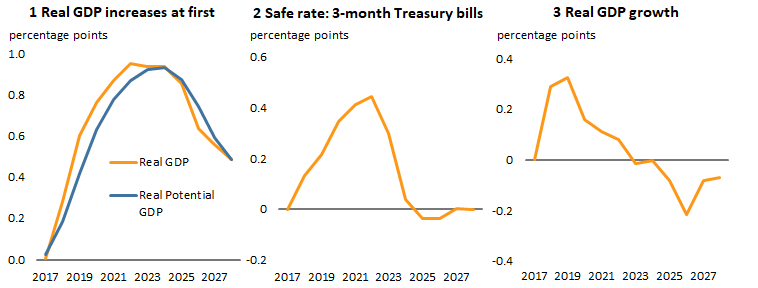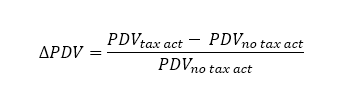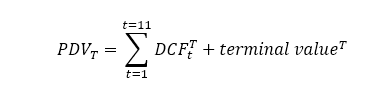# CBO and Markets Agree: The 2017 US Tax Act Will Not Raise Stock Prices Much

May 4, 2018 11:30 AM
Photo Credit:
REUTERS/Brendan McDermid

The Congressional Budget Office (CBO) forecasts a relatively small increase in US GDP over the next decade resulting from recent tax legislation. In its April 2018 outlook, the CBO estimates that the 2017 Tax Cuts and Jobs Act (TCJA) passed last year will put the real GDP level on average 0.7 percent higher than it otherwise would be over the next 10 years, a minor effect. This forecast implies that the TCJA only accounts for a modest 3.6 percent increase in stock prices, as increases in dividends will be partly offset by future increases in the 3-month Treasury bill interest rate (the safe rate) in present value terms. This blog post suggests that CBO forecasts for the effects of the TCJA are in line with market expectations, in that they both expect low tax multipliers in the current business cycle.

Figures 1 and 2 show the impact of the TCJA on baseline CBO forecasts for the GDP level and the safe rate, respectively. According to the CBO, the TCJA first increases real GDP relative to potential GDP through demand effects (figure 1), driving the safe rate up by 0.4 percentage point by 2022. But the CBO also expects the TCJA to increase the level of potential GDP “by boosting investment and labor,” justifying a somewhat lower safe rate at the end of the decade. The economic effect of the TCJA would peak between 2018 and 2020 with a yearly impact of 0.3 percentage point on real GDP growth (figure 3). After 2023, the economic effect of the TCJA on growth would turn negative. Ten years from now, real GDP would only be 0.5 percentage point higher than it would otherwise be (figure 1).

Economic effects of the 2017 TCJA according to CBOTCJA = Tax Cuts and Jobs Act of 2017
Sources: Congressional Budget Office (CBO) and author's calculations.

CBO forecasts imply that the TCJA will have a modest impact on the US economy and therefore on stock prices. There are three effects to consider here: The TCJA will have a one-time leveling effect on post-tax earnings given pre-tax earnings, the growth impact of TCJA will increase the long-term flow of dividends, and the change in the safe rate will alter the rate of return required by investors. As for the last two effects, CBO forecasts imply that the present discounted value (PDV) of firms exposed to the US market will change thus:The CBO provides forecasts for the interest and growth rates, with no tax act andwith the tax act, on an 11-year horizon. Supposing that dividends move one-for-one with real GDP over the long run and that the cost of capital moves one-for-one with the safe rate, CBO forecasts pin down future cash flows for the next 11 years. The present value of future dividends in a given tax regime T is expressed by:Where DCF is the discounted cash flows computed using the standard formula. Two problems remain, however, before computing the change in PDV. First, the PDV of post-horizon forecasts, or terminal value, must be considered and requires assumptions for the post-horizon growth and interest rates. Here, the assumption is that the tax act has no long-run effect on growth and interest rates after the forecast horizon, so the change in terminal value only comes from base effects. The long-run values for the growth and interest rates are assumed to be equal to CBO forecasts for the last period of the forecast horizon. Second, the computation of a PDV requires the use of a discount rate equal to the safe rate plus an equity risk premium X. Taking an equity premium of 5 percent (Campbell 2008) and abstracting from the one-off effect on earnings for now, the CBO forecasts imply that PDV falls by 1 percent—a marginal decrease in stock prices. Looking at the contribution of the growth and safe rates separately, the growth effect generates an increase of 0.5 percent in PDV, dwarfed by the cost of capital effect, which is –1.5 percent. The intuition behind this result is that the CBO forecasts a larger change in the interest rate than in the growth rate over the forecast horizon.

But the TCJA will also generate a one-off increase in after-tax earnings given pre-tax earnings of 4 percent (Blanchard et al. 2018), creating a shift in the path of future dividends that needs to be accounted for. This effect will be partly offset by the two other effects so that total effect of the TCJA would be an increase of 3 percent in PDV. If this small increase in PDV is at odds with the large increase in stock prices observed after the US election, much of the recent increase in stock prices was in fact not generated by the TCJA. To isolate the effect of the TCJA on stock prices, Blanchard et al. (2018) regresses changes in S&P 500 prices on the changes in probability of passage of the TCJA, controlling for the world business cycle. The authors find that the TCJA can account for a 2.5 to 5 percent increase in PDV. The CBO implied change in PDV therefore lies in the range of estimated market price reactions. In other words, CBO and market expectations are relatively well aligned, although the CBO is possibly on the lower side.

Why are expectations so low despite the expected high fiscal costs of the TCJA? Cohen-Setton et al. (2018a) show that fiscal stimuli are dependent on the state of the economy, as fiscal multipliers tend to be lower in good times, when the economy is not in recession or at the end of a business cycle. When the economy is close to potential, fiscal spending usually generates inflation, which the Fed, given its inflation mandate, usually offsets with contractionary policy. The markets and CBO forecasts appear to have indeed factored in the state-dependency of fiscal multipliers in estimating the long-run effect of the TCJA.

Author's note: I thank Olivier Blanchard and Jérémie Cohen-Setton for very helpful comments.

## References

Blanchard, Olivier, Christopher G. Collins, Mohammad R. Jahan-Parvar, Thomas Pellet, and Beth Anne Wilson. 2018. Why Has the Stock Market Risen So Much Since the US Presidential Election? PIIE Policy Brief 18-4 (February). Washington: Peterson Institute for International Economics.

Campbell, John Y. 2008. Estimating the equity premium. Canadian Journal of Economics 41(1): 1–21.

Cohen-Setton, Jérémie, Egor Gornostay, and Colombe Ladreit de Lacharrière. 2018a (forthcoming). Large Fiscal Expansions in OECD Countries: Identification and Effects. PIIE Working Paper. Washington: Peterson Institute for International Economics.

Cohen-Setton, Jérémie, Egor Gornostay, and Colombe Ladreit de Lacharrière. 2018b. Impact of the Trump Fiscal Stimulus on US Economic Growth. PIIE Realtime Economic Issues Watch (April 6). Washington: Peterson Institute for International Economics.

CBO (Congressional Budget Office). 2018. The Budget and Economic Outlook: 2018 to 2028. April. Washington.

## Notes

1. Real GDP grew on average at 2.6 percent, while real dividends grew at 2.4 percent on the 1980–2014 period.

2. This happens to be a relatively mild assumption as growth and safe rate effects for the last year of the forecast horizon are already close to zero.

3. Results are not very sensitive to the equity premium. For X=4% and X=6%, the change in PDV is -1 percent and -0.9 percent respectively.